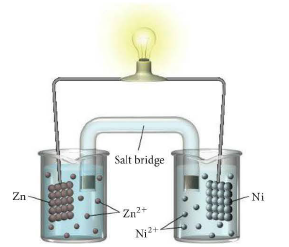# Problem: Consider the molecular view of an electrochemical cell involving the overall reaction: Zn(s) + Ni2+(aq) → Zn2+(aq) + Ni(s) Draw a similar sketch of the cell after it has generated a substantial amount of electrical current.

###### FREE Expert Solution
80% (474 ratings)
###### Problem Details

Consider the molecular view of an electrochemical cell involving the overall reaction:
Zn(s) + Ni2+(aq) → Zn2+(aq) + Ni(s)

Draw a similar sketch of the cell after it has generated a substantial amount of electrical current.What scientific concept do you need to know in order to solve this problem?

Our tutors have indicated that to solve this problem you will need to apply the Galvanic Cell concept. You can view video lessons to learn Galvanic Cell. Or if you need more Galvanic Cell practice, you can also practice Galvanic Cell practice problems.

What is the difficulty of this problem?

Our tutors rated the difficulty ofConsider the molecular view of an electrochemical cell invol...as medium difficulty.

How long does this problem take to solve?

Our expert Chemistry tutor, Jules took 2 minutes and 46 seconds to solve this problem. You can follow their steps in the video explanation above.

What professor is this problem relevant for?

Based on our data, we think this problem is relevant for Professor Jones' class at University of Guelph.

What textbook is this problem found in?

Our data indicates that this problem or a close variation was asked in Chemistry: A Molecular Approach - Tro 3rd Edition. You can also practice Chemistry: A Molecular Approach - Tro 3rd Edition practice problems.